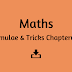Mathematics is an important subject that is included in almost every competitive exam. Preparing for math can be challenging, especially if you don't have a solid understanding of the concepts and formulas. However, with the help of some simple tricks and formulas, you can solve math problems quickly and easily. In this article, we will share some important maths tricks and formulas for competitive exams along with a downloadable PDF.

Tricks for Competitive Exams:

1. Multiplication by 11: To multiply any two-digit number by 11, just add the two digits together and place the result in the middle. For example, 11 x 35 = 385.

2. Squaring any two-digit number ending in 5: To square a two-digit number ending in 5, multiply the first digit by itself plus 1, and add 25 to the result. For example, 65^2 = (6x7)25 = 4225.

3. Finding the unit digit: To find the unit digit of a number raised to any power, just look for the pattern in the unit digits of the first few powers. For example, the unit digit of 7^1 is 7, the unit digit of 7^2 is 9, the unit digit of 7^3 is 3, and so on. So, to find the unit digit of 7^26, we just need to look for the pattern in the unit digits, which is 7, 9, 3, 1. Since 26 is an even number, the unit digit of 7^26 is 9.

4. Divisibility rules: To quickly check if a number is divisible by another number, there are some simple rules to follow. For example, a number is divisible by 2 if its last digit is even, a number is divisible by 3 if the sum of its digits is divisible by 3, and so on.

Formulas for Competitive Exams:

1. Area of a triangle: A = 1/2 x base x height

2. Perimeter of a rectangle: P = 2(length + width)

3. Volume of a cylinder: V = πr^2h, where r is the radius of the base and h is the height of the cylinder.

4. Simple Interest: I = (P x R x T)/100, where P is the principal amount, R is the rate of interest, and T is the time period.

Conclusion:

Mathematics is a crucial subject for most competitive exams, and it is essential to have a good understanding of the concepts and formulas. By using the tricks and formulas mentioned above, you can save time and solve math problems quickly and easily. Make sure to practice these tricks and formulas regularly to improve your math skills and score well in competitive exams.

Maths Tricks for Competitive Exams & Formulas PDF DownloadReviewed by SSC NOTES on October 15, 2023 Rating: 5